# CHAPTER 1- Vectors

In very basic terms, a vector can be thought of as an arrow in the Euclidean plane. This arrow has a starting (initial ) point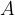$A$ and an ending (final) point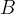$B$:This vector will be represented as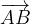$\overrightarrow {AB}$. We thus see that a vector has two quantities associated with it:
 (a) a magnitude (b) a direction
These two quantities are necessary to carry someone from$A$ to$B$; these two quantities are sufficient to uniquely specify a vector.
Contrast a vector with a scalar, which is a physical quantity with just a magnitude but no associated direction.
Think of a force acting on a block.To specify this force, you must specify both its magnitude and direction, and thus force is a vector quantity. You might, as an example, specify this force by saying that it is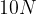$10 N$ strong and is applied at an angle of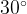${30^ \circ }$ to the horizontal.
On the other hand, think about the work done by this force over a certain distance, which is obviously a scalar since it will be a quantity with just a magnitude and no direction.
In the discussion that follows, we will see that for a physical quantity to be classified as a vector, it must satisfy another constraint in addition to possessing a magnitude and a direction: it must satisfy the vector law of addition section –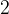$2$. In fact, there do exist quantities (like the rotation of a rigid body) which posses both magnitude and direction but are not vectors because they do not satisfy the addition law.
We can represent a vector using its end-points (like$\overrightarrow {AB}$ earlier) or we can use lower-case letters (like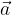$\vec a$). For any vector$\vec a$, we have three associated characteristics:
Length
The length (or magnitude) of$\vec a$ will be denoted by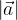$\left| {\vec a} \right|$. Length is obviously a scalar.
Support
This is the line along which the vector$\vec a$ lies
Sense
The vector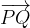$\overrightarrow {PQ}$ will have a sense from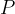$P$ to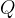$Q$ along the support of$\overrightarrow {PQ}$, while that of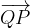$\overrightarrow {QP}$ will be from$Q$ to$P$ along the support of$\overrightarrow {PQ}$ . Thus, the sense of a vector specifies its direction along its support.
Some more terminology is in order before we begin to see the properties of vectors:
(A) Zero vectors:
A vector of magnitude zero is called a zero vector and is denoted as$\vec 0$. A zero vector does not really have any direction, since how can you define the direction of a point? We thus assume a zero vector to have any arbitrary direction. In a sense, you may say that the zero vector is not a proper vector. In fact, vectors other than the zero vector are called proper vectors!
(B) Unit vectors:
Vector$\vec a$ is a unit vector if it is of unit length, i.e, if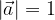$\left| {\vec a} \right| = 1$. If$\vec a$ is a unit vector, it is generally denoted as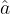$\hat a$.
(C) Collinear vectors:
These are essentially parallel vectors, i.e, have the same or parallel support.
Some elaboration must be done here: we will encounter, in our study of this chapter, either fixed vectors or free vectors. As the name suggests, a fixed vector has its absolute position fixed with respect to any choosen coordinate system; a free vector is one which can be translated to any position in space, keeping its magnitude and direction fixed.
For example, suppose that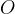$O$ is the origin and$A$ is a fixed point in the coordinate system. Then the vector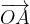$\overrightarrow {OA}$ is fixed because its starting point,$O$ is fixed.
On the other hand, suppose a vector$\vec a$ corresponds to going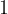$1$ unit right and$2$units up in the coordinate system. Then$\vec a$ is free since it can be translated to anywhere in the coordinate system; it will still represent going$1$ unit right and$2$units up.
When we talk of collinear vectors, it is implied that the vectors being talked about are free vectors. Thus, for two vectors to be collinear, their supports only need to be parallel (and not necessarily the same).
(D) Equal vectors:
Two vectors$\vec a$ and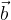$\vec b$ are equal if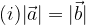$(i) |\vec a| = |\vec b|$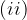$(ii)$ their directions are the same, i.e. their supports are the same OR parallel, and they have the same sense.
It should be evident that when we are saying that two vectors are equal, we implicitly assume that the we talking about free vectors.
(E) Co-initial vectors:
Fixed vectors having the same initial point are called co-initial vectors
(F) Co-terminus vectors:
Fixed vectors having the same ending point are called co-terminus vectors.
(G) Co-planar vectors:
A system of free vectors is coplanar if their supports are parallel to the same plane.
Note that defined this way, two free vectors will always be coplanar. This is because you can always bring these two vectors together to have the same initial point, and then a plane can always be drawn through the two vectors. On the other hand, three free vectors might or might not be coplanar; let us think of this more elaborately. Assume three free vectors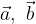$\vec a,\,\,\vec b$ and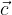$\vec c$. Suppose you bring together$\vec a$ and$\vec b$ to have the same initial point$O$; you then draw the plane passing through$\vec a$ and$\vec b$. Now, when$\vec c$ is translated so that its initial point is$O$, it is not necessary for$\vec c$ also to lie in the plane that you drew through$\vec a$ and$\vec b$. Thus,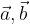$\vec a,\vec b$ and$\vec c$ might or might not be coplanar(H) Negative of a vector:
The negative of a vector$\vec a$, denoted by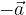$- \vec a$, is a vector with the same magnitude as$\vec a$ but has exactly the opposite direction.
(I) Position vector:
The position vector of a point$P$ is a fixed vector which joins the origin of the reference frame to the point$P$.
We’ll be using position vectors a lot in our later discussions.

### Related Posts

There is no other posts in this category.